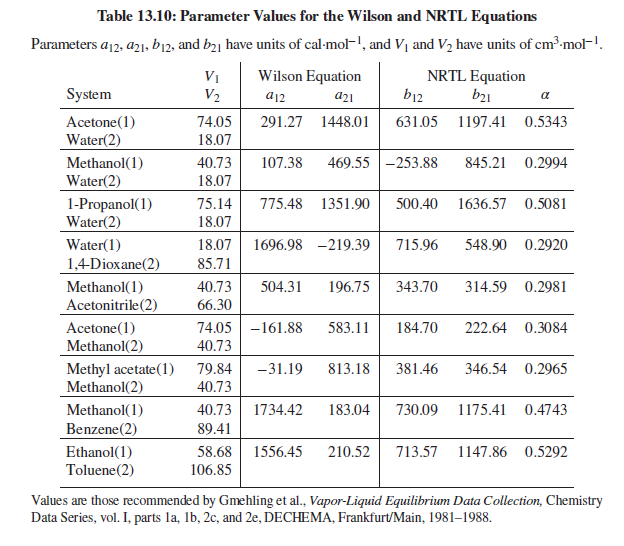×
Get Full Access to Introduction To Chemical Engineering Thermodynamics - 8 Edition - Chapter 13 - Problem 13.53
Get Full Access to Introduction To Chemical Engineering Thermodynamics - 8 Edition - Chapter 13 - Problem 13.53

×ISBN: 9781259696527 2049

## Solution for problem 13.53 Chapter 13

Introduction to Chemical Engineering Thermodynamics | 8th Edition

• Textbook Solutions
• 2901 Step-by-step solutions solved by professors and subject experts
• Get 24/7 help from StudySoup virtual teaching assistantsIntroduction to Chemical Engineering Thermodynamics | 8th Edition

4 5 1 378 Reviews
11
5
Problem 13.53

Problems 13.43 through 13.54 require parameter values for the Wilson or NRTL equation for liquid-phase activity coefficients. Table 13.10 gives parameter values for both equations. Antoine equations for vapor pressure are given in Table B.2, Appendix B.For the acetone(1)/methanol(2)/water(3) system, based on Eq. (13.19) and the Wilson equation, make the following calculations:

(a) $$B U B L T: P=101.33 \mathrm{kPa}, x_{1}=0.3, x_{2}=0.4$$.

(b) $$D E W T: P=101.33 \mathrm{kPa}, y_{1}=0.3, y_{2}=0.4$$.

(c) P, T − flash : $$P=101.33 \mathrm{kPa}, T=\frac{1}{2}\left(T_{\text {bubble }}+T_{\text {dew }}\right), z_{1}=0.3, z_{2}=0.2$$.

Text Transcription:

BUBLT: P=101.33 kPa, x_1=0.3, x_2=0.4

DEW T: P=101.33 kPa, y_1=0.3, y_2=0.4

P=101.33 kPa, T=1/2(T_bubble+T_dew), z_1=0.3, z_2=0.2

Step-by-Step Solution:
Step 1 of 3

Lecture 3: Graphics Problem Solving  Review Engineer's Scale Practice Sheet Outcomes  Use an engineering scale to scale features of a drawing  Generate graphic solutions of problems  Practice legible writing Exercise 1  Construct a triangle of side lengths 3 cm, 4 cm, and 5 cm and measure the internal angles with your protractor. Use a scale of 1" = 1 cm. Cannot use calculator. o Tools  Engineering scale  Compass  Protractor Exercise 2  Graphically solve the 4 sided polygon to scale on engineering paper  Choose a scale so that your drawing is within the solid border and covers 1/2- 3/4 of your page  Measure and report length of sides A & B. Measure angle α then check your answer mathematically. Lettering  Standards exist for lettering and numbering on drawings  Students with poor penmanship should practice until they consistently print legibly Suggested problems 1 A right triangle has a hypotenuse length of 52 inches and an angle of 52 degrees. What are the measurements for the 3rd angle and the length of the two legs Check your answer mathematically. 2 Draw a right triangle that has leg measurements of 41 mm and 26 mm. From your drawing, measure the length of the hypotenuse and the two unknown angles. Check your answer mathematically. Lecture 4: Orthographic Drawings Outcomes  Explain orthographic views (the glass box)  Identify first and third angle projections 

Step 2 of 3

Step 3 of 3

## Discover and learn what students are asking

Unlock Textbook Solution